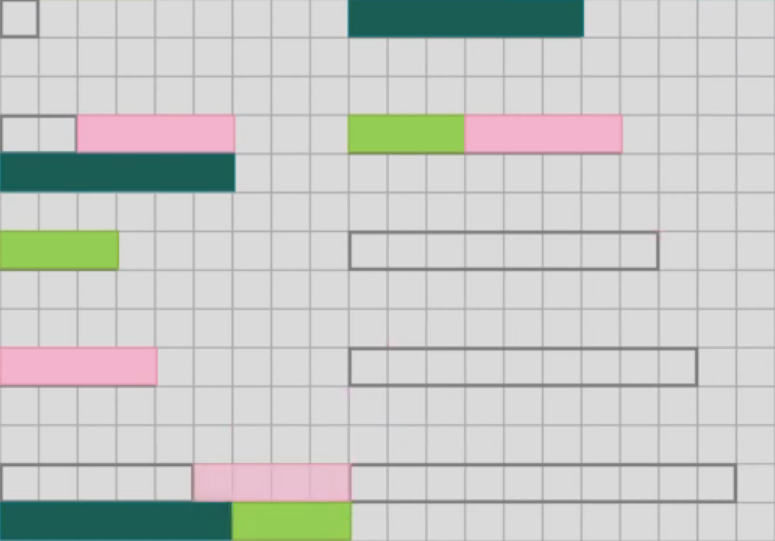#### You may also like### Pair Sums

Five numbers added together in pairs produce: 0, 2, 4, 4, 6, 8, 9, 11, 13, 15 What are the five numbers?### Summing Consecutive Numbers

15 = 7 + 8 and 10 = 1 + 2 + 3 + 4. Can you say which numbers can be expressed as the sum of two or more consecutive integers?### Big Powers

Three people chose this as a favourite problem. It is the sort of problem that needs thinking time - but once the connection is made it gives access to many similar ideas.

# Combining Lengths

##### Age 11 to 16Challenge Level
If you have not used the Cuisenaire Environment before, you might find it helpful to look at the instructions and video before trying this problem.

Start by choosing three different colours of rod.
The challenge is to try to make as many different lengths between 1 and 10 as you can without using any rod more than once.

For example, you might choose light green (3), pink (4) and dark green (6).
In the picture below, you can see how these rods can be combined to make lengths of 2, 3, 4, 5, 6 and 7.There are some other lengths we can make, but it's not possible to make 8 with these three rods. If you were allowed to use a rod twice, you could use two pinks together to make 8, but you are only allowed to use each rod once.

Choose three rods of your own, and see which lengths you can make with them.
Can you find a set of three rods that you can use to make any length from 1 to 10?

It's possible to find a set of rods that measures even more than ten different lengths.
Can you find a set of three rods that you can use to make any length from 1 to 11? Or 1 to 12?
What's the furthest that you can go?

Is there only one set of three rods that allows you to measure the largest number of consecutive lengths?

What if you could use a set of four rods?...

Is there a way to decide how long the rods should be for any set of rods?

Is there a quick way to work out how far a set of rods will allow you to measure?

What would happen if you could only add lengths together, and couldn't subtract?